Physics 15/10/2018

# Units in GCSE Physics

By KEVIN QUINN

Without units, science wouldn’t make sense. Units allow us to use numbers to describe the world. That’s why maths is called the language of science. Understanding units and using them correctly can make all the difference in the exams. You want to nail those maths-heavy questions in your GCSE Physics papers? Then you need to be at one with your units. This blog explains how.

## Physical quantities

A physical quantity is anything that can be measured and represented by a number.

Base units are the fundamental measures that are used to describe all physical quantities. You have to know six of them for the science GCSEs, as described below: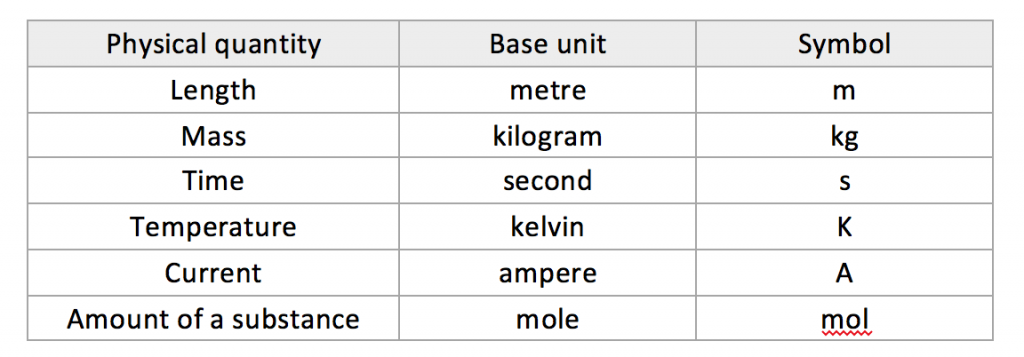All other physical quantities can be represented by combinations of base units which are called derived units.  Examples of derived units include m2, m3, kg/m3 and m/s2. Below, we’ll discuss the most important derived units and provide a few tips to push up your marks in the GCSE exams.

## Degrees Celsius (°C)

The base unit for temperature is the kelvin (K), but its derived unit is the degree Celsius (°C).  We can use the following simple equation to convert between these scales: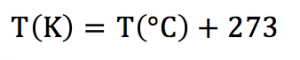The lowest possible temperature is called absolute zero. This is the point at which the particles that make up a substance stop vibrating completely. Absolute zero is a temperature of 0 K or -273 °C.

## Power

In physics, power can be defined in one of two ways: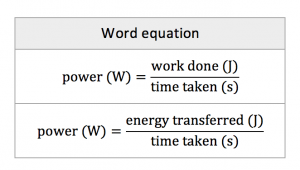Look at the right hand sides of each of these equations. In both cases, the power (in watts, W) is calculated by dividing something in joules (J) by something in seconds (s). This means that an equivalent unit for the watt is the joule per second: 1 W = 1 J/s. In questions on power, even if you forget both of the above equations, remembering that 1 W = 1 J/s can help you in getting to the correct answer.

EXAMPLE.  The power adaptor of a laptop is labelled ‘50 W’. Calculate the amount of electrical energy which is converted by the adaptor per hour.

SOLUTION.  Even if you have a nightmare mind-blank in the exam and forget both the above equations, this question is very doable if you remember that 50 W = 50 J/s.  In other words, 50 J of electrical energy are converted by the adaptor every second.  To calculate the amount of energy converted in one hour, we just have to multiply 50 J by the number of seconds in one hour, which leads us to the final answer of 50 × (60 × 60) = 180,000 J (or 180 kJ).

## Knowing and understanding units

There’s a big difference between understanding a unit and just knowing it. For example, you might remember that you can use the unit gram per cubic centimetre (g/cm3) for the density of something, but you might have forgotten the actual equation which you have to use to calculate it. How then could you tackle the question below?

EXAMPLE. A block of aluminium of mass 135 g has a volume of 50 cm3. Use this information to calculate the density of aluminium.

The answer is in the unit of g/cm3. This density is calculated by dividing something in grams (g) by something in cubic centimetres (cm3), in other words, by dividing a mass by a volume.  So the correct equation to use must be: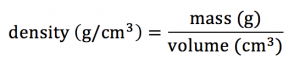which means that the correct answer can be calculated as follows: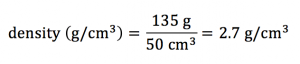There’s another big tip for picking up marks on questions like this: if you’re not sure what to do next in a mathematical question, check the answer line. Most questions (not all) will include the unit on the answer line. So in this case, if g/cm3 is written next to the blank space where the answer is meant to go, that’s a clear signal that to do the calculation you need to divide a mass by a volume.

## Speed distance and time

The last section of this blog is on the units of speed and velocity. The most common of these is the metre per second (m/s). Again, do your best to read and fully understand this unit every time you see it in the exam.  If we say that something is moving at so many metres per second, it means that it covers that distance every second. So the correct equation for the average speed of the object must be: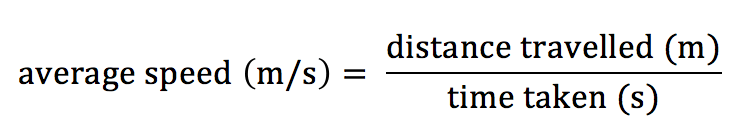That may seem fairly simple, but how would you tackle the question below?

EXAMPLE. A speedboat is travelling at a constant speed of 54 km/h.  Calculate the distance (in metres) it will travel in one minute.

SOLUTION. This question is a bit tricky at first sight. We know that 1 minute is equal to 60 seconds, so if we knew the speed of the boat in metres per second (m/s), we could easily use the above equation to calculate the distance travelled in metres. But we don’t – instead the speed has been given in kilometres per hour (km/h).

The boat is travelling at 54 km/h, so it would cover a distance of 54 km if it kept moving at this speed for a full hour. As 1 km = 1000 m, the boat is travelling at a rate of 54,000 metres per hour (m/h). We know that it will travel a much shorter distance than this in one second, as there are (60 × 60 = 3600) seconds in one hour. To convert the speed of 54,000 m/h into metres per second (m/s) then, we simply divide 54,000 by 3,600 which gives an answer of 15. In other words, a speed of 54 km/h is equivalent to a speed of 15 m/s.

(Note, as you get comfortable with the maths, you can convert from km/h to m/s in a single step, by multiplying 54 by 1000 ÷ 3600).

From this point, the final answer can easily be calculated as follows:

distance (m) = speed (m/s) x time (s)

distance (m) = 15 x 60 = 900m

Kevin Quinn